# VBA Course: Controls (Part 2)

## Option Buttons (OptionButton)

Unlike checkboxes, the user can only select one option button per group.

Here, we will separate the option buttons into 2 groups and save the results in 2 cells:The file: userform3.xlsm

The first step is to create the button groups (as for now, you can only select one answer from the 8 options).

To do this, select the first 4 controls and enter a value in the GroupName property: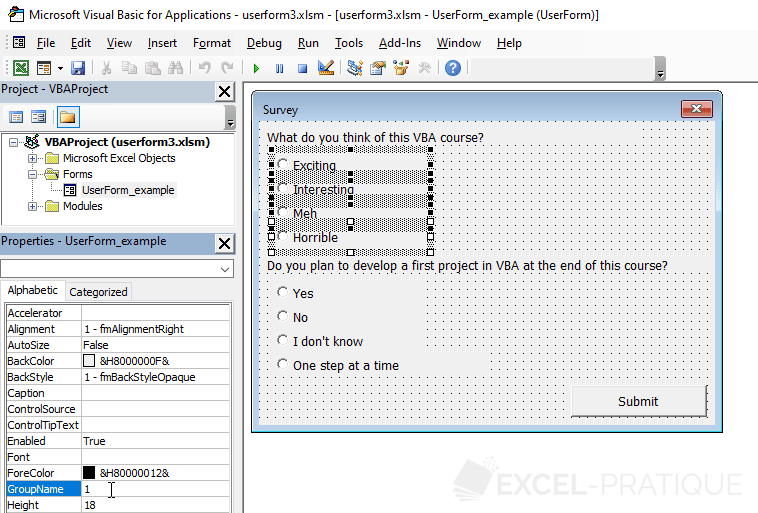Repeat the same process for the other 4 controls (entering a different value).

You can now select one answer per group.

To save the responses in the worksheet cells, we will first add the Click event of the Submit button.

Then, we need to add a loop for each group of option buttons and save the information when the control's value is True:

``````Private Sub CommandButton_submit_Click()

Dim i As Integer

'Question 1
For i = 1 To 4
If Controls("OptionButton_a_" & i) Then Range("A2") = Controls("OptionButton_a_" & i).Caption
Next

'Question 2
For i = 1 To 4
If Controls("OptionButton_b_" & i) Then Range("B2") = Controls("OptionButton_b_" & i).Caption
Next

'Close

End Sub``````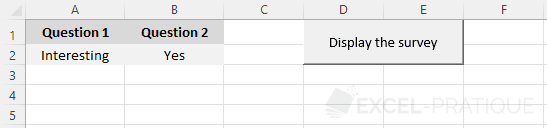Instead of saving the choice as text, let's save its number (from 1 to 4):

``````Private Sub CommandButton_submit_Click()

Dim i As Integer

'Question 1
For i = 1 To 4
If Controls("OptionButton_a_" & i) Then Range("A2") = i
Next

'Question 2
For i = 1 To 4
If Controls("OptionButton_b_" & i) Then Range("B2") = i
Next

'Close

End Sub``````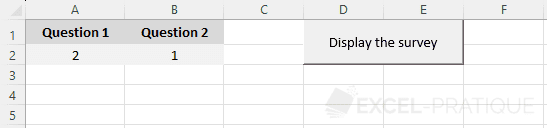If we want to prevent the form from being saved unless the user has answered both questions, one solution is to save the choice of each group in a variable, then check if there is a choice for both variables, and save the choices in the cells:

``````Private Sub CommandButton_submit_Click()

Dim i As Integer, choice1 As Integer, choice2 As Integer

'Question 1
For i = 1 To 4
If Controls("OptionButton_a_" & i) Then choice1 = i
Next

'Question 2
For i = 1 To 4
If Controls("OptionButton_b_" & i) Then choice2 = i
Next

'If 2 responses
If choice1 > 0 And choice2 > 0 Then

'Save
Range("A2") = choice1
Range("B2") = choice2

'Close

'If one or more missing responses
Else

'Error message
MsgBox "You must answer all the questions before submitting the form.", 48, "Error"

End If

End Sub``````

The file: userform3b.xlsm

## Dropdown List (ComboBox) and List Box (ListBox)

Here is the starting point of this new example: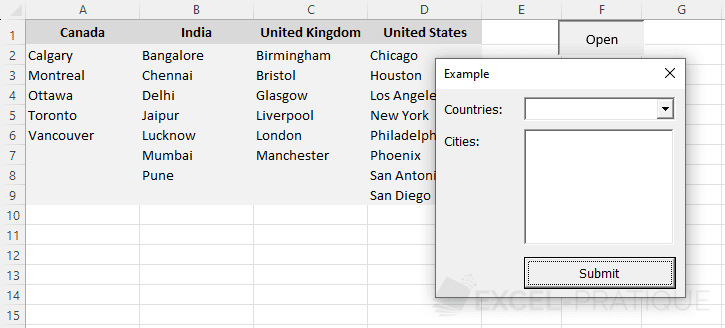The file: userform4.xlsm

When the UserForm is launched, we want the 4 countries to be loaded into the dropdown list (using the AddItem method):

``````Private Sub UserForm_Initialize()

Dim i As Integer

'Loop to add the 4 countries to the dropdown list
For i = 1 To 4
Next

End Sub``````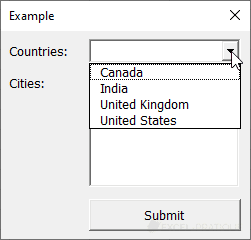When the selection changes in the dropdown list, the corresponding list of cities should be displayed in the list box.

To do this, we need to know the column number as well as the number of cities in that column.

The ListIndex property of the dropdown list corresponds to the index number of the selection (unlike the Value property, which corresponds to the text value).

Knowing that ListIndex starts at 0 (like arrays), the column number is:

``column = ComboBox_countries.ListIndex + 1``

To get the number of rows in the chosen country column, we can search for the row number of the last non-empty cell in a block of cells, like this:

``numRows = Cells(1, column).End(xlDown).Row``

With this information, we can now create the Change event of the dropdown list:

``````Private Sub ComboBox_countries_Change()

Dim column As Integer, numRows As Integer

'Clear the list box (otherwise the cities are added in addition to the previous ones)
ListBox_cities.Clear

'Index number of the selection
column = ComboBox_countries.ListIndex + 1

'If the column number = 0 (meaning no country selected), exit the procedure
If column = 0 Then Exit Sub

'Number of rows in the chosen country column
numRows = Cells(1, column).End(xlDown).Row

'Loop to add the cities to the list box
For i = 2 To numRows
Next

End Sub``````

Finally, we just need to add a click event to the Submit button to process this information. In this case, we'll simply display the selection in a message box:

``````Private Sub CommandButton_submit_Click()

MsgBox "Selected City: " & ListBox_cities.Value

End Sub``````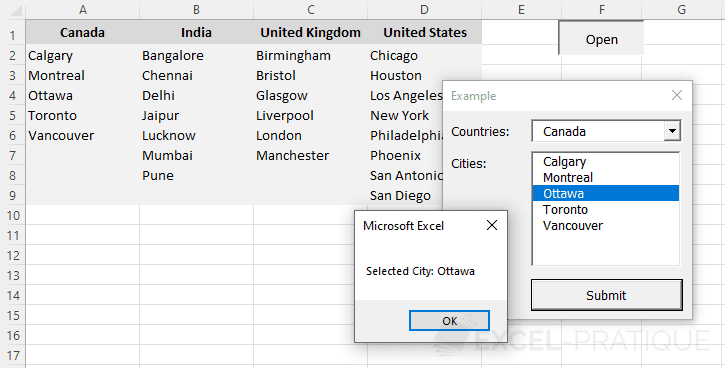The file: userform4b.xlsm